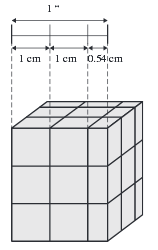# Problem: One inch is equal to 2.54 cm. The below image shows a line that is 1 in long with centimeter segments drawn on the line. A cube that is 1 in on each side with lines on each face of the cube that are 1 cm apart is also shown.How many cubic centimeters are there in 1 in3?

###### FREE Expert Solution

We’re being asked to convert 1 cm3 into in3.

To do so, we need the following conversion factors:

1 in = 2.54 cm

81% (305 ratings)###### Problem Details

One inch is equal to 2.54 cm. The below image shows a line that is 1 in long with centimeter segments drawn on the line. A cube that is 1 in on each side with lines on each face of the cube that are 1 cm apart is also shown.How many cubic centimeters are there in 1 in3?

Frequently Asked Questions

What scientific concept do you need to know in order to solve this problem?

Our tutors have indicated that to solve this problem you will need to apply the Dimensional Analysis concept. You can view video lessons to learn Dimensional Analysis. Or if you need more Dimensional Analysis practice, you can also practice Dimensional Analysis practice problems.

What professor is this problem relevant for?

Based on our data, we think this problem is relevant for Professor TBA's class at Thomas Jefferson University.